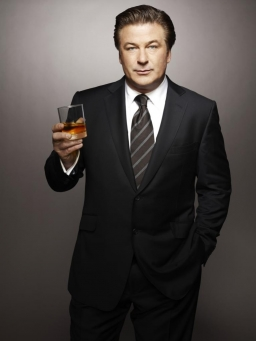# Buttons

Men's shirt has sewn 9 buttons and blouse 3 buttons. Together they sewed 60 pieces and consumed 390 buttons. How many shirts?

Result

k =  35

#### Solution:

60=k+h
390= 9k+3h

h+k = 60
3h+9k = 390

h = 25
k = 35

Calculated by our linear equations calculator.Our examples were largely sent or created by pupils and students themselves. Therefore, we would be pleased if you could send us any errors you found, spelling mistakes, or rephasing the example. Thank you!

Leave us a comment of this math problem and its solution (i.e. if it is still somewhat unclear...):Be the first to comment!Tips to related online calculators
Do you have a linear equation or system of equations and looking for its solution? Or do you have quadratic equation?

## Next similar math problems:

1. Football seasonDalibor and Adam together scored 97 goals in the season. Adam scored 9 goals more than Dalibor. How many goals scored each?
2. BlackberriesDaniel, Jolana and Stano collected together 34 blackberries. Daniel collected 8 blackberries more than Jolana, Jolana 4 more than Stano. Determine the number blackberries each collected .
3. MushroomsEva and Jane collected 114 mushrooms together. Eve found twice as much as Jane. How many mushrooms found each of them?
4. Family parcelsIn father will he divided the land so that the older son had three bigger part than younger son. Later elder son gave 2.5 ha field to younger and they had both the same. Determine the area of family parcel.
5. SummerjobThree students participated in the summerjob. Altogether they earn 1780, -. Peter got a third less than John and Paul got 100,- more than Peter. How much got every one of them?
6. Fifth of the numberThe fifth of the number is by 24 less than that number. What is the number?
7. Vijuviju has 40 chickens and rabbits. If in all there are 90 legs. How many rabbits are there with viju??
8. Dining roomThe dining room has 11 tables (six and eight seats). In total there are 78 seats in the dining room. How many are six-and eight-seat tables?
9. Three brothersThe three brothers have a total of 42 years. Jan is five years younger than Peter and Peter is 2 years younger than Michael. How many years has each of them?
10. Three figures - numbersThe sum of three numbers, if each is 10% larger than the previous one, is 662. Determine the figures.
11. TreesAlong the road were planted 250 trees of two types. Cherry for 60 CZK apiece and apple 50 CZK apiece. The entire plantation cost 12,800 CZK. How many was cherries and apples?
12. Substitutionsolve equations by substitution: x+y= 11 y=5x-25
13. The largerThe larger of two numbers is nine more than four times the smaller number. The sum of the two numbers is fifty-nine. Find the two numbers.
14. SchoolsThree schools are attended by 678 pupils. To the first attend 21 students more and to the third 108 fewer students than to second school. How many students attend the schools?
15. Rabbits 3Viju has 40 chickens and rabbits. If in all there are 90 legs. How many rabbits are there with Viju?
16. EquationsSolve following system of equations: 6(x+7)+4(y-5)=12 2(x+y)-3(-2x+4y)=-44
17. ATC campThe owner of the campsite offers 79 places in 22 cabins. How many of them are triple and quadruple?